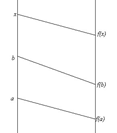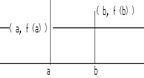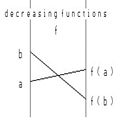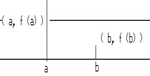CHAPTER III Applications of the Derivative (Draft- 2014 work in progress)
III.B. First Derivative Analysis
Draft Version 4/19/14

In Chapter I.G  we looked informally at some of the possible analysis based on the first and second derivatives. In that work we relied on the sense that this analysis made in the interpretations and the visualizations of some simple examples. In the next two sections we will review that analysis with a more examples providing further justification for the bases of this type of analysis.

Review of an informal approach: Traveling along a road on the way from San Francisco to Los Angeles we usually watch our trip mileage change and use this to estimate how much distance remains before we reach our destination. The mileage numbers get larger as time passes, and the distance to Los Angeles is diminishing. If we were to treat the distance traveled as a function of time, it should make sense to claim that our speed is the derivative of this function, that it is positive, and that this is connected directly to the fact that the distance traveled is increasing. The distance remaining till Los Angeles can also be treated as a function, and its derivative will be the same in magnitude as our speed, but opposite in sign, as this distance is diminishing as we proceed to our destination. As we saw in Chapter I.G, it appears informally from the interpretation that a positive derivative indicates an increasing function while a negative derivative is evidence for a function that has decreasing values.

Differential Estimates:
If you use the differential to estimate the values of a function $f$ close to a point a where the derivative $f '(a)$ is positive and $x$ is larger than $a$, it should make sense that $f(x)$ will be larger than $f(a)$. Likewise, if the derivative is negative then the differential estimate suggests the value of $f(x)$ will be less than $f(a)$. These very simple and sensible situations point again to the importance of knowing the sign of the derivative to determine whether the values of the function are increasing or decreasing close to a point in the domain.
Of course with the ever increasing graphing capabilities of computers and hand held calculators, the role of the calculus in visualizing the graph of a function has changed. Before the technology of graphing was available, if variables satisfied some function relationship then information about the derivative would be useful to draw a quick sketch of the graph to visualize the relationship. Now the graphing technology supplies this visualization without much effort or thought in many situations. The calculus today provides the background that explains in some sense why we see what we see while the technology supplies the graph. It also can alert us to features of the graph that the technology may have missed. The problems of scaling and the basic nature of graphing technology can only provide the illusion of a complete picture because it only samples the values of the function to create its pictures. In this section we will apply the ability to find and analyze the derivative of a function to gain information that explains the behavior of that function. From this we will be able to gain further insight into the extremes of the function. We begin the work of the section with some definitions, then we'll state and apply the major results, and finally we'll justify the major results using an important theorem in the theoretical structure of the calculus called the Mean Value Theorem.

III.B.1. Increasing and Decreasing Functions.
The description of a function as increasing or decreasing should be familiar to you. We include the more formal definition at this stage for some review. Suppose $f$ is defined for all points in an interval $I$ and $a$ and $b$ are any two points in $I$

Definition. Suppose $f$ is defined for all points in an interval $I$ and $a$ and $b$ are any two points in $I$.
We say that $f$ is (strictly) increasing on the interval $I$ if $a < b$ implies $f(a) < f(b)$ for all possible choices of $a$ and $b$ in the interval.
We say that $f$ is non-decreasing on the interval $I$ if $a < b$ implies $f(a) \le f(b)$ for all possible choices of $a$ and $b$ in the interval.
We say that $f$ is (strictly) decreasing on the interval $I$ if $a < b$ implies $f(a) > f(b)$ for all possible choices of $a$ and $b$ in the interval.
We say that $f$ is non-increasing on the interval $I$ if  $a < b$ implies $f(a) \ge f(b)$ for all possible choices of $a$ and $b$ in the interval.

Interpretations: 1. In the motion interpretation an increasing function is always moving in an upward direction on the mapping figure. Arrows drawn between the source and target on an interval where the function is increasing will never cross each other in the region between the source and the target. See Figure 1.Figure 1Figure 2.Figure 3Figure 4
Non-decreasing functions may remain at the same value on the target for some time interval, but once they pass above a value in the target they never return to it in an interval where they are non-decreasing.
2. In the graphical interpretation an increasing function on an interval has a graph which has points higher on the graph as the points move from left to right. Once the graph lies above or on any horizontal line, it will not be found below it or even at the same level to the right of that point while over the interval. See Figure 2.
The graphs of non-decreasing functions may appear in some sections like horizontal lines, but they may not appear below the level of a horizontal line at a point to the right of any point at which that level is reached.
3. The first definitions apply precisely to the type of function that arises as a (cumulative) probability distribution function $F$ from a random variable $X$. Recall that such a function is defined at $A$ by finding the probability that $X \le A$. If $A < B$, certainly $F(A) \le F(B)$ since the likelihood of $X$ being less than or equal to $B$ is certainly no less than that of $X$ being less than or equal to $A$.
4.    An economic interpretation of an increasing function might consider how costs increase with increased levels of production. On the other hand the annual salary of many employees can be seen as non decreasing functions of time.
5.    Similar interpretations are possible for the concepts of decreasing and non-increasing, but are left for the reader to make.
See Figures 3 and 4.
__________________________________________________________________________________________________________________________
The following two key results relate the derivative to increasing/non-decreasing behavior of a function in a way which should seem familiar and make sense at this stage:
 Theorem III.B.1. (P) If $f$ is a differentiable function on an interval $I$ with $f '(x) > 0$ for all $x$ in the interval, then $f$ is increasing on the interval. If $f '(x) \ge 0$ for all $x$ in the interval, then $f$ is non-decreasing on the interval. Theorem III.B.2. (N) If $f$ is a differentiable function on an interval $I$ with $f '(x) < 0$ for all $x$ in the interval, then $f$ is decreasing on the interval. If $f '(x)\le 0$ for all $x$ in the interval, then $f$ is non-increasing on the interval.

___________________________________________________________________________________________________________________________
Here is an example of the application of these two theorems to analyze a function's behavior. Notice that the analysis uses the continuity of the derivative function with the intermediate value theorem to determine the intervals where the function is increasing and decreasing.

Example III.B.1.
Find any intervals of the real numbers for which the function $f(x) = x^4-8x^2$ is increasing. For which intervals is the function decreasing?
Solution: By the previous theorems we need to find those intervals where $f '(x) > 0$ and those for which $f '(x) < 0$. We first find that $f '(x) = 4 x^3 - 16 x$ and determine the critical points, i.e., where $f '(x) = 0$. These are $x = -2, 0,$ and $2$. Since $f '(x)$ is a continuous function, an application of the intermediate value theorem (Chapter I.I.B. ) shows that the sign of $f '(x)$ can change only at one of the critical points. Testing the value of $f '$ at non-critical points will determine whether the derivative is positive or negative between the critical points. With some simple calculations you can see easily that $f '(10) > 0, f '(-10) < 0, f '(-1) > 0,$ and $f '(1) < 0$. Thus the derivative is positive for the intervals $(-2,0)$ and $(2,\infty)$ and is negative for the intervals $(-\infty,-2)$ and $(0,2)$. We visualize this situation in the following sign chart figure for $f'$.
 $f '(x)$  - - - - - 0 + + + + + + 0 - - - - - - 0 + + + + +                                                                                         $x$                 -2        -1         0      1       2       3      4 Figure 5: Sign Chart for $f'(x)$

Based on this analysis of the derivative we can say now that the function $f$ is increasing on the intervals $(-2,0)$ and $(2,\infty)$ and is decreasing for the intervals $(-\infty,-2)$ and $(0,2)$. Note as well at this stage the values of the function at the critical points are $f (-2) = -16$,  $f (0) = 0$, and $f (2) = -16$. We can combine this information with the previous figure to arrive at an enhanced sign chart figure showing the full analysis of the function using the first derivative.
[Increasing is indicated by $\nearrow$ while decreasing is indicated by $\searrow$.]

 $f '(x)$  - - - - - 0 + + + + + + 0 - - - - - - 0 + + + + +                                                                                         $x$                 -2        -1          0      1       2       3      4 $f$ is:  $\searrow$   -16    $\nearrow$          0     $\searrow$   -16         $\nearrow$ Figure 6: Enhanced Sign Chart for $f'(x)$

The results of this analysis are illustrated in the graph of $f$ as shown in GeoGebra graph and mapping diagram and are consistent with the values of the function shown in the accompanying table on Figure 7.
 Figure 7

Example III.B.2. Let $f (x) = x \ln(x)$ with $x>0$. Find any intervals for which this function is increasing.

Solution: Our analysis begins finding $f '(x) = \ln(x) + 1$. Again the crucial piece of work is to find the critical points. Solving $0 = \ln(x) + 1$ we see that $f '(x) = 0$ only when $\ln(x)= -1$, i.e., when $x=\frac 1e$. Using intermediate value theorem analysis for the derivative again allows us to see what the sign of the derivative is on the intervals determined by this critical point.
We have $f '(.1) = \ln(.1)+1 < 0$  and $f '(1) = \ln(1) + 1 = 1$, so the derivative is positive only on the interval $(\frac 1e,\infty )$ as Figure 8 illustrates. Applying Theorem P we see that $f$ is increasing on the interval $(\frac 1e, \infty)$. See Figure 8 and Figure 9.

 $f '(x)$  - - - - - 0 + + + + + + + + + + + + + + +                                                                                        $x$    0       $\frac 1e$   .5      1                          2   $f$ is:     $\searrow$ $-\frac 1e$  $\nearrow$                                   Figure 8: Enhanced Sign Chart for $f'(x)$

 Figure 9

Example III.B.3. Let $f(x) = \sin(x) + \cos(x)$. Find any intervals between $0$ and $2\pi$ for which this function is increasing.
Solution:
Our analysis begins by finding the derivative, $f '(x) = \cos(x) - \sin(x)$. Again the crucial piece of work is to find the critical points. Solving $0 = \cos(x) - \sin(x)$ we see that $f '(x) = 0$ only when $\sin(x) = \cos(x)$, i.e., when $x = \pi/4$ and $5\pi/4$. Using intermediate value theorem analysis for the derivative again allows us to see what the sign of the derivative is on the intervals determined by these critical points. We can see that $f '(0)=1, f '(\pi) = -1$, and $f '(2\pi) = 1$, so the derivative is positive only on the intervals $[0,\pi/4)$ and $(5\pi/4, 2\pi]$ as Figure *** illustrates.
Applying Theorem P we see that $f$ is increasing on the intervals $[0,\pi/4)$ and $(5\pi/4, 2\pi]$. See Figures 10 and 11.

 $f '(x)$ + + + + 0 - - - - - - - - 0 + + + + + + +                                                                                       $x$     0        $\pi/4$          $5\pi/4$         $2\pi$               $f$ is:     $\nearrow$ $\sqrt 2$  $\searrow$     $-\sqrt 2$ $\nearrow$                 Figure 10: Enhanced Sign Chart for $f'(x)$

 Figure 11

Exercises III.B.1:

 Generic GeoGebra for First Derivative Analysis Exercises.